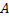# Statement 1: The internal angle bisector of angleof a trianglewith sidesandasand, respectively isStatement 2: Image of pointwith respect tolies on sideof the triangle a) Statement 1 is True, Statement 2 is True; Statement 2 is correct explanation for Statement 1 b) Statement 1 is True, Statement 2 is True; Statement 2 is not correct explanation for Statement 1 c) Statement 1 is True, Statement 2 is False d) Statement 1 is False, Statement 2 is True

## Question ID - 54540 :- Statement 1: The internal angle bisector of angleof a trianglewith sidesandasand, respectively isStatement 2: Image of pointwith respect tolies on sideof the triangle a) Statement 1 is True, Statement 2 is True; Statement 2 is correct explanation for Statement 1 b) Statement 1 is True, Statement 2 is True; Statement 2 is not correct explanation for Statement 1 c) Statement 1 is True, Statement 2 is False d) Statement 1 is False, Statement 2 is True

3537

(b)

Bisectors of angleareorandAccording to given equations of sides, internal angle bisector atwill have negative slope. Also, image ofwill lie onrespect to both bisectors, from which we can conclude thatis internal angle bisector. Hence, statement 2 is not correct explanation of statement 1

Next Question :
 Statement 1: Each point on the lineis equidistant from the linesStatement 2: The locus of a point  which is equidistant from two given lines is the angular bisector of two the lines a) Statement 1 is True, Statement 2 is True; Statement 2 is correct explanation for Statement 1 b) Statement 1 is True, Statement 2 is True; Statement 2 is not correct explanation for Statement 1 c) Statement 1 is True, Statement 2 is False d) Statement 1 is False, Statement 2 is True